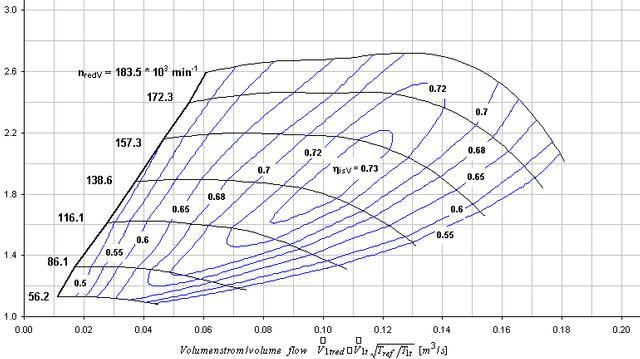# Compressor map

(diff) ← Older revision | Latest revision (diff) | Newer revision → (diff)
Pressure ratio (y-axis)
The absolute outlet pressure divided by the absolute inlet pressure.
Mass air flow rate (x-axis)
The mass of air flowing through the intake/compressor over time. Usually lbs/min or CFM.
Surge line
The left hand boundary of the map, where the air flow is too low for the current pressure ratio. Blow off valves or Bypass valves are often used to relieve excess pressure to prevent sudden off throttle induced pressure surges.
Choke line
The right hand boundary of the map, where the turbo is approaching its flow limit
Overspeed line
The top boundary of the map, where the turbo is approaching its maximum shaft speed. Past this point, the turbo is liable to "overspin" and cause catastrophic bearing failure.
Turbo speed lines
The lines of constant turbo shaft speed.
Efficiency islands
The concentric regions on the map representing compressor efficiency. The smallest island in the center of the map is the "sweet spot", or where turbo is the most efficient.

## Pressure Ratio

Expressed asor, more commonly,Where

•is the absolute pressure at the turbo compressor outlet
•is the absolute pressure at the turbo compressor inlet
•is pressure at the throttle body inlet
•is ambient air pressure
•is intercooler pressure drop (pressure difference betweenand)
•is intake pressure drop (pressure difference betweenand)

### Examples

Google calculator provides a handy way to deal with unit issues.

For example, with 19psi boost, 1psi IC drop, and 1psi inlet drop, type this into google (assuming you live at sea level):

(1 atm + 19 psi + 1 psi)/(1 atm - 1 psi)=

and you get:

A few others:

As you can see, as ambient pressure drops (e.g. higher elevation) or intake/IC drops increase, your pressure ratio increases along with it.

## Boost from Pressure Ratio

To calculate boost from desired pressure ratio:For example, with 2.5 PR, 1psi IC drop, and 1psi inlet drop, type this into google (assuming you live at sea level):

2.5 * (1 bar - 1 psi) - 1 bar - 1 psi in psi

and you get:

As you can see, as ambient pressure drops (e.g. higher elevation) or intake/IC drops increase, your boost limit (for a given PR limit) decreases along with it.

## Air Flow

Most turbo maps express air flow in terms of mass per time (commonly grams per second or lbs per minute) or volume per time (commonly cubic feet per second, CFM, or cubic meters per second.)

If you have accurate MAF numbers (i.e. your car is not modded in a way that scales the MAF readings), you can convert this directly to the desired units.

If you do not have accurate MAF numbers, but do have an approximate horsepower target, you can use the rule of thumb that 1 lb/min of air generates 10 horsepower.

### Examples

#### From g/sec

To convert grams per second into pounds per minute, divide by 7.55

To convert grams per second into m3/sec, divide by 1225 (density of air at sea level)

300 / 1225 = 0.2449 (300 (grams per second)) / ((1 225 g) per (cubic meter)) = 0.2449 m3 / s

If you have two turbos, divide g/sec by twice that, or 2450

300 / 2450 = 0.1224

#### From horsepower

To convert horsepower to pounds per minute, divide by 10.

To convert horsepower to m3/sec, divide by 1620

420 / 1620 = 0.259

If you have two turbos, divide horsepower by twice that, or 3240

420 / 3240 = 0.130

## Plotting(to be filled in with data above)